# How to use DynForest with survival outcome?

## Introduction to pbc2 dataset

We use DynForest on the pbc2 dataset (Murtaugh et al. 1994) to illustrate our methodology. Data come from the clinical trial conducted by the Mayo Clinic between 1974 and 1984. For the illustration, we consider a subsample of the original dataset resulting to 312 patients and 7 predictors. Among these predictors, the level of serum bilirubin (serBilir), aspartate aminotransferase (SGOT), albumin and alkaline were measured at inclusion and during the follow-up leading to a total of 1945 observations. Sex, age and the drug treatment were collected at the enrollment. During the follow-up, 140 patients died before transplantation, 29 patients were transplanted and 143 patients were alive. The time of first event (alive or any event) was considered as the event time. We aim to predict in this illustration the death without transplantation on patients suffering from primary billiary cholangitis (PBC) using clinical and socio-demographic predictors, considering the transplantation as a competing event.

## Managing data

To begin, we load DynForest package and pbc2 data and we split the subjects into two datasets: (i) one dataset to train the random forest using $$2/3$$ of patients; (ii) one dataset to predict on the other $$1/3$$ of patients.

library("DynForest")
set.seed(1234)
id <- unique(pbc2$id) id_sample <- sample(id, length(id)*2/3) id_row <- which(pbc2$id%in%id_sample)
pbc2_train <- pbc2[id_row,]
pbc2_pred <- pbc2[-id_row,]

Then, we build the dataframe in the longitudinal format (i.e. one observation per row) for the longitudinal predictors including: id the unique patient identifier; time the observed time measurements; serBilir, SGOT, albumin and alkaline the longitudinal predictors. We also build the dataframe with the time-fixed predictors including: id the unique patient identifier; age, drug and sex predictors measured at enrollment. The nature of each predictor needs to be properly defined with as.factor() function for categorical predictors (e.g. drug and sex).

timeData_train <- pbc2_train[,c("id","time",
"serBilir","SGOT",
"albumin","alkaline")]
fixedData_train <- unique(pbc2_train[,c("id","age","drug","sex")])

The first step aims to build the random forest using the DynForest() function. We need to specify the mixed model of each longitudinal predictor through a list containing the fixed and random formula for the fixed effect and random effects of the mixed models, respectively. To allow for a flexible trajectory over time, splines can be used in formula using splines package.

timeVarModel <- list(serBilir = list(fixed = serBilir ~ time,
random = ~ time),
SGOT = list(fixed = SGOT ~ time + I(time^2),
random = ~ time + I(time^2)),
albumin = list(fixed = albumin ~ time,
random = ~ time),
alkaline = list(fixed = alkaline ~ time,
random = ~ time))

Here, we assume a linear trajectory for serBilir, albumin and alkaline, and quadratic trajectory for SGOT. For this illustration, we build the outcome object containing a list with type set to surv (for survival data) and Y contains a dataframe in wide format (one subject per row) with: id the unique patient identifier; years the time-to-event data; event the event indicator.

Y <- list(type = "surv",
Y = unique(pbc2[,c("id","years","event")]))

## Build the random forest

We build the random forest using DynForest() function with the following code:

res_dyn <- DynForest(timeData = timeData_train,
fixedData = fixedData_train,
timeVar = "time", idVar = "id",
timeVarModel = timeVarModel, Y = Y,
ntree = 200, mtry = 3, nodesize = 2, minsplit = 3,
cause = 2, seed = 1234)

In a survival context with multiple events, it is also necessary to specify the event of interest with the argument cause. We thus fixed cause = 2 to specify the event of interest (i.e. the death event). For the hyperparameters, we arbitrarily chose mtry = 3, nodesize = 2 and minsplit = 3.

Overall information about the random forest can be output with summary() function. The summary for the random forest in our illustration is displayed below:

summary(res_dyn)

DynForest executed with survival (competing risk) mode
Splitting rule: Fine & Gray statistic test
Out-of-bag error type: Integrated Brier Score
Leaf statistic: Cumulative incidence function
----------------
Input
Number of subjects: 208
Longitudinal: 4 predictor(s)
Numeric: 1 predictor(s)
Factor: 2 predictor(s)
----------------
Tuning parameters
mtry: 3
nodesize: 2
minsplit: 3
ntree: 200
----------------
----------------
DynForest summary
Average depth by tree: 6.61
Average number of leaves by tree: 28.01
Average number of subjects by leaf: 4.71
Average number of events of interest by leaf: 1.91
----------------
Out-of-bag error based on Integrated Brier Score
Out-of-bag error: Not computed!
----------------
Time to build the random forest
Time difference of 3.000176 mins
---------------- 

We executed DynForest() function in survival mode with competing events. In this mode, we use the Fine & Gray statistic test for the splitting rule and the cumulative incidence function (CIF) for the leaf statistic. To build the random forest, we included 208 subjects with 4 longitudinal (Longitudinal), 1 continuous (Numeric) and 2 categorical (Factor) predictors. The summary() function also returns some statistics about the trees. For instance, we have on average 4.7 subjects and 1.9 death events by leaf. The number of subjects by leaf should always be higher than nodesize hyperparameter. OOB error should be first computed using compute_OOBerror() function (see section below) to be displayed on summary output.

To further investigate the process in each tree, the split details for the tree 1 can be output with the following code:

head(res_dyn$rf[,1]$V_split)

type id_node var_split feature   threshold   N Nevent depth
1 Longitudinal       1         3       1 -0.21993804 129     49     1
2 Longitudinal       2         2       1  5.57866304  26     21     2
3      Numeric       3         1      NA 61.83057715 103     28     2
4 Longitudinal       4         2       3  1.42021938  18     13     3
5       Factor       5         1      NA          NA   8      8     3
6 Longitudinal       6         3       2 -0.01010312  92     22     3
tail(res_dyn$rf[,1]$V_split)

type id_node var_split feature threshold N Nevent depth
48         Leaf     192        NA      NA        NA 4      2     8
49         Leaf     193        NA      NA        NA 2      2     8
50         Leaf     194        NA      NA        NA 2      1     8
51 Longitudinal     195         4       1 -27.58024 4      3     8
52         Leaf     390        NA      NA        NA 2      1     9
53         Leaf     391        NA      NA        NA 2      2     9

For instance for the interpretation of the node split, the subjects were split at node 1 (id_node) using the first random-effect (feature = 1) of the third Longitudinal predictor (var_split = 3) with threshold = -0.2199. The predictor name can be found using the predictor number in timeData and fixedData datasets. Therefore, the subjects at node 1 with albumin values below to -0.2199 drop in node 2, otherwise in node 3. Another example with the leaves, 4 subjects are included in the 192, and among them 2 subjects have the event of interest.

Estimated cumulative incidence function (CIF) for a single tree can be displayed using Y_pred element of rf. For instance, the CIF of the cause of interest for leaf 192 can be displayed using the following code:

plot(res_dyn$rf[,1]$Y_pred[]$2, type = "l", col = "red", xlab = "Years", ylab = "CIF", ylim = c(0,1)) However, CIF computed on a single tree is not relevant. It should also be computed over all trees of the random forest. For a subject, estimated CIF over the random forest is obtained by averaging the tree-specific CIF of the leaf where the subject belongs. For instance, for subject 104, we display in figure 1 the tree-specific CIF for the 9 first trees where this subject is used to grow the trees. This figure shows how the estimated CIF can be differ across the trees and requires to be averaged as they are obtained on a few subjects from the leaves in which subject 104 is assigned.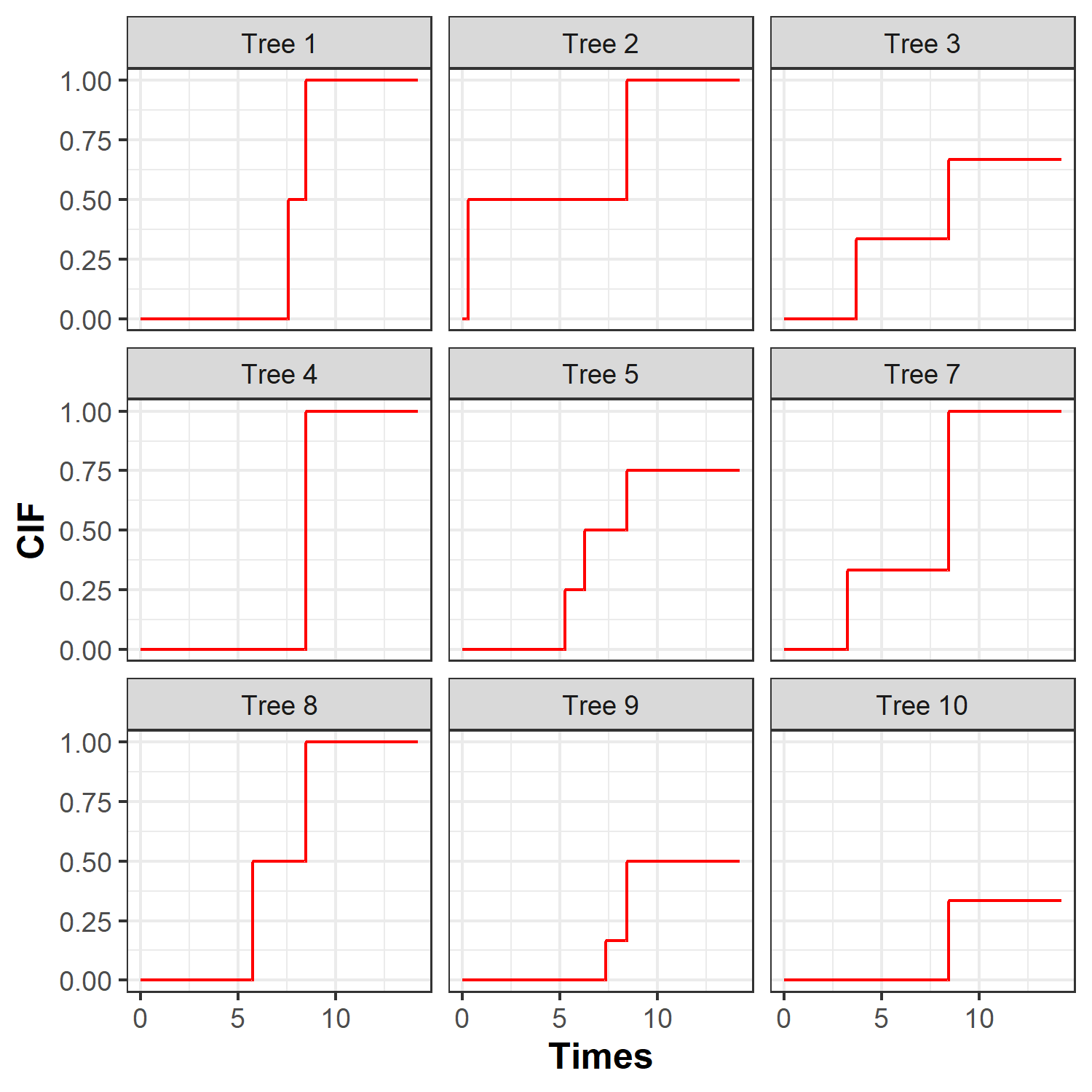Figure 1: Estimated cumulative incidence functions of death before transplantation for subject 104 over 9 trees. ## Out-Of-Bag error The Out-Of-Bag error (OOB) aims at assessing the prediction abilities of the random forest. It is computed using compute_OOBerror() function with a DynForest class object as main argument, such as: res_dyn_OOB <- compute_OOBerror(DynForest_obj = res_dyn) compute_OOBerror() returns the OOB error by individual (oob.err) and the overall OOB error for the random forest can be obtained by averaging the OOB error. mean(res_dyn_OOB$oob.err)

 0.1238627

In a survival context, the OOB error is evaluated using the Integrated Brier Score (IBS) (Gerds and Schumacher 2006). We obtain an IBS of 0.124 computed from time 0 to the maximum event time. The time range can be modified using IBS.min and IBS.max arguments to define the minimum and maximum, respectively. To maximize the prediction ability of the random forest, we want to minimize the OOB error. This can be done by tuning the hyperparameters. OOB error can also be displayed using summary() function applied to the returning object.

## Predict the outcome

The software allows to predict the outcome for a new subject using the trained random forest. Dynamic predictions can be computed by fixing a prediction time (called landmark time) from which prediction is made using all the history of the individual up to this landmark time (including the longitudinal and time-fixed predictors).

For the illustration, we only select the subjects still at risk at the landmark time of 4 years. We build the dataframe for those subjects and we predict the individual specific CIF using predict() function as follows:

id_pred <- unique(pbc2_pred$id[which(pbc2_pred$years>4)])
pbc2_pred_tLM <- pbc2_pred[which(pbc2_pred\$id%in%id_pred),]
timeData_pred <- pbc2_pred_tLM[,c("id","time",
"serBilir","SGOT",
"albumin","alkaline")]
fixedData_pred <- unique(pbc2_pred_tLM[,c("id","age","drug","sex")])
pred_dyn <- predict(object = res_dyn,
timeData = timeData_pred,
fixedData = fixedData_pred,
idVar = "id", timeVar = "time",
t0 = 4)

predict() function provides several elements. To get more graphical results, the plot_CIF() function can be used to display the CIF of death before transplantation for given subjects. For instance, we computed the CIF for subjects 102 and 260 with the following code and displayed them on the figure 2.

plot_CIF(DynForestPred_obj = pred_dyn,
id = c(102, 260))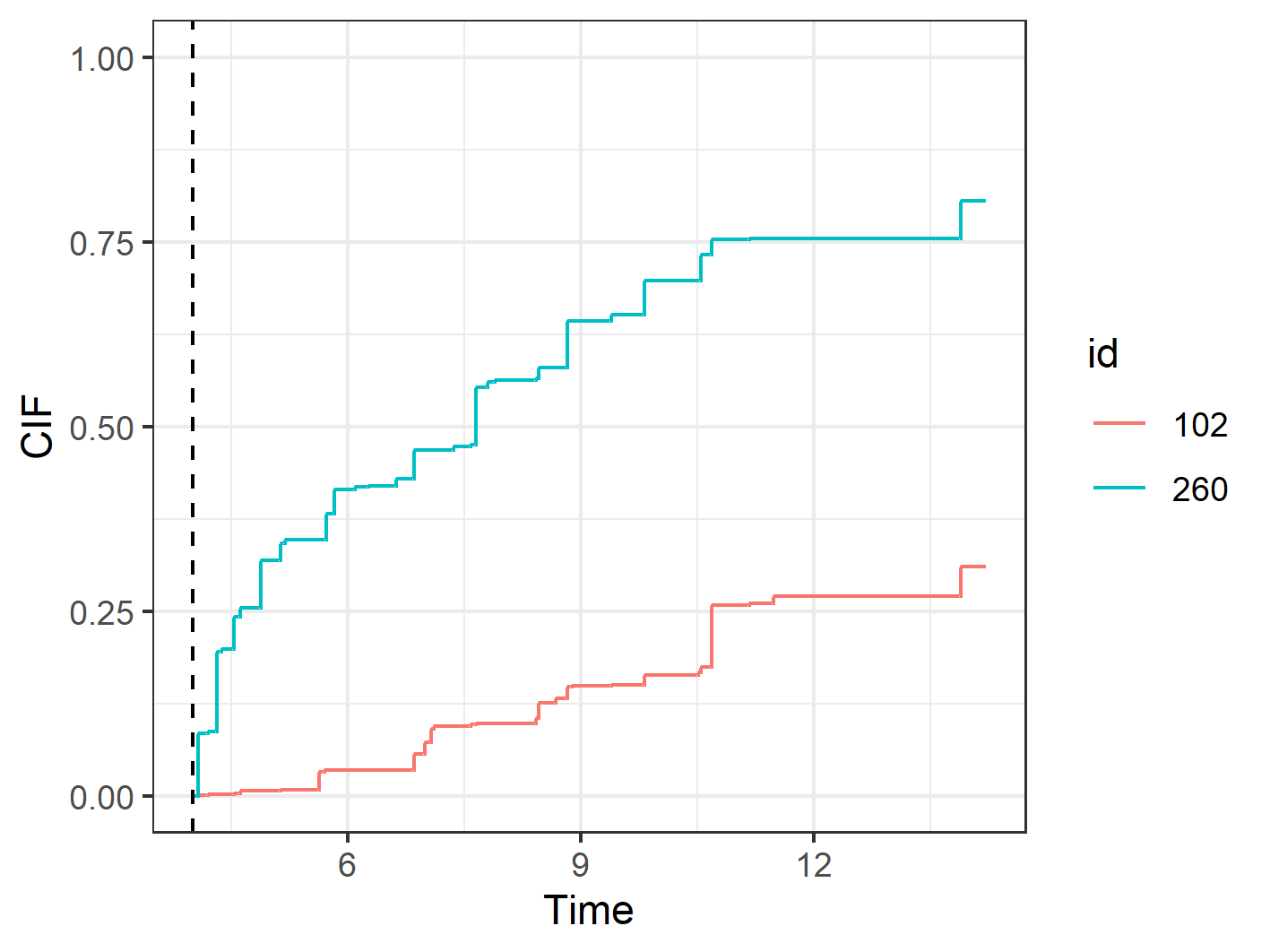Figure 2: Predicted cumulative incidence function for subjects 102 and 260 from landmark time of 4 years (represented by a dashed line).

The first year after the landmark time (at 4 years), we observe a rapid increase of the risk of death for subject 260 compared to subject 102. We also notice that after 10 years from landmark time, subject 260 has a probability of death almost three times higher that the one of subject 102.

## Explore the most predictive variables

### Variable importance

The main objective of the random forest is to predict an outcome. But usually, we are interested to identify which predictors are the most predictive. The VIMP statistic can be computed using compute_VIMP() function. This function returns the VIMP statistic for each predictor with Importance element. These results can also be displayed using plot() function in percentage with PCT argument set to TRUE.

res_dyn_VIMP <- compute_VIMP(DynForest_obj = res_dyn, seed = 123)
plot(x = res_dyn_VIMP, PCT = TRUE)

The VIMP results are displayed in figure 3A. The most predictive variables are serBilir, albumin and age with the largest VIMP percentage. Without serBilir, the OOB error of prediction was reduced by 28%.

In the case of correlated predictors, the predictors can be regrouped into dimensions and the VIMP can be computed at the dimension group level with the gVIMP statistic. Permutation is done for each variable of the group simultaneously. The gVIMP is computed with the compute_gVIMP() function. This function has the group argument to define the group of predictors as a list. For instance, with two groups of predictors (named group1 and group2), the gVIMP statistic is computed using the following code:

group <- list(group1 = c("serBilir","SGOT"),
group2 = c("albumin","alkaline"))
res_dyn_gVIMP <- compute_gVIMP(DynForest_obj = res_dyn,
group = group, seed = 123)
plot(x = res_dyn_gVIMP, PCT = TRUE)

Similar to VIMP statistic, the gVIMP results can be displayed using plot() function. The figure 3B shows that group1 has the highest gVIMP percentage with 32%.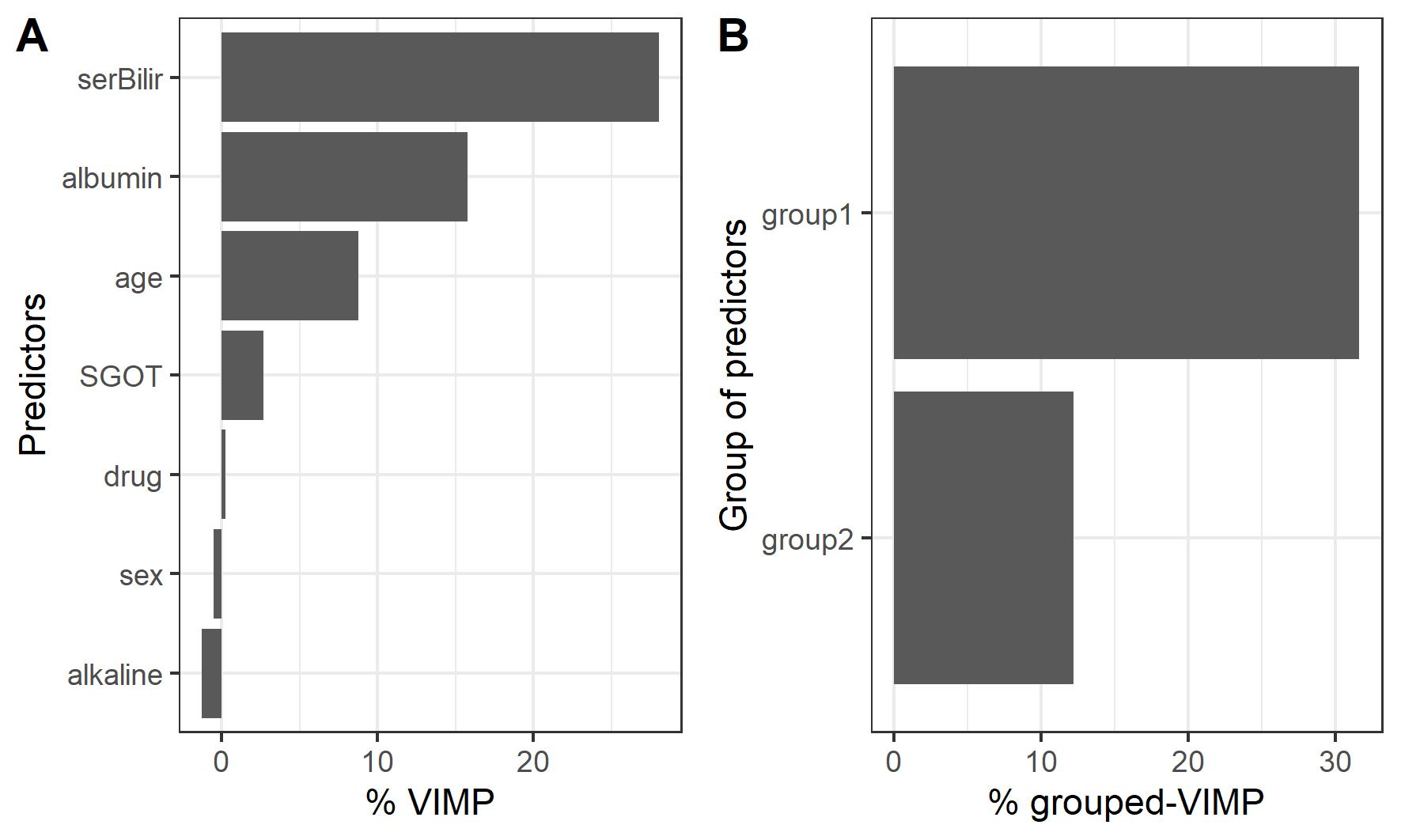Figure 3: Using VIMP statistic (A), we observe that serBilir and albumin are the most predictive predictors. Using grouped-VIMP statistic (B), group1 (serBilir and SGOT) has more predictive ability than group2 (albumin and alkaline).

To compute the gVIMP statistic, the groups can be defined regardless of the number of predictors. However, the comparison between the groups may be harder when group sizes are very different.

### Minimal depth

To go further into the understanding of the tree building process, the var_depth() function extracts useful information about the average minimal depth by feature (min_depth), the minimal depth for each feature and each tree (var_node_depth), the number of times that the feature is used for splitting for each feature and each tree (var_count).

Using an object from var_depth() function, plot() function allows to plot the distribution of the average minimal depth across the trees. plot_level argument defines how the average minimal depth is plotted, by predictor or feature.

res_dyn_max <- DynForest(timeData = timeData_train,
fixedData = fixedData_train,
timeVar = "time", idVar = "id",
timeVarModel = timeVarModel, Y = Y,
ntree = 200, mtry = 7, nodesize = 2, minsplit = 3,
cause = 2, seed = 1234)
depth_dyn <- var_depth(DynForest_obj = res_dyn_max)
plot(x = depth_dyn, plot_level = "predictor")
plot(x = depth_dyn, plot_level = "feature")

The distribution of the minimal depth level is displayed in figure 4 by predictor and feature. Note that the minimal depth level should always be interpreted with the number of trees where the predictor/feature is found. Indeed, to accurately appreciate the importance of a variable minimal depth, it has to be part of the candidates at each node. This is why we strongly advice to compute the minimal depth on random forest with mtry hyperparameter chosen at its maximum.

In our example, we ran a random forest with mtry hyperparameter set to its maximum (i.e. mtry = 7) and we computed the minimal depth on this random forest. We observe that serBilir, albumin and age have the lowest minimal depth, indicating these predictors are used to split the subjects at early stage in 200 out of 200 trees, i.e 100% (figure 4A). The minimal depth level by feature (figure 4B) provides more advanced details about the tree building process. For instance, we can see that the random-effects for serBilir (indicating by bi0 and bi1 on the graph) are the earliest features used on 198 and 194 out of 200 trees, respectively.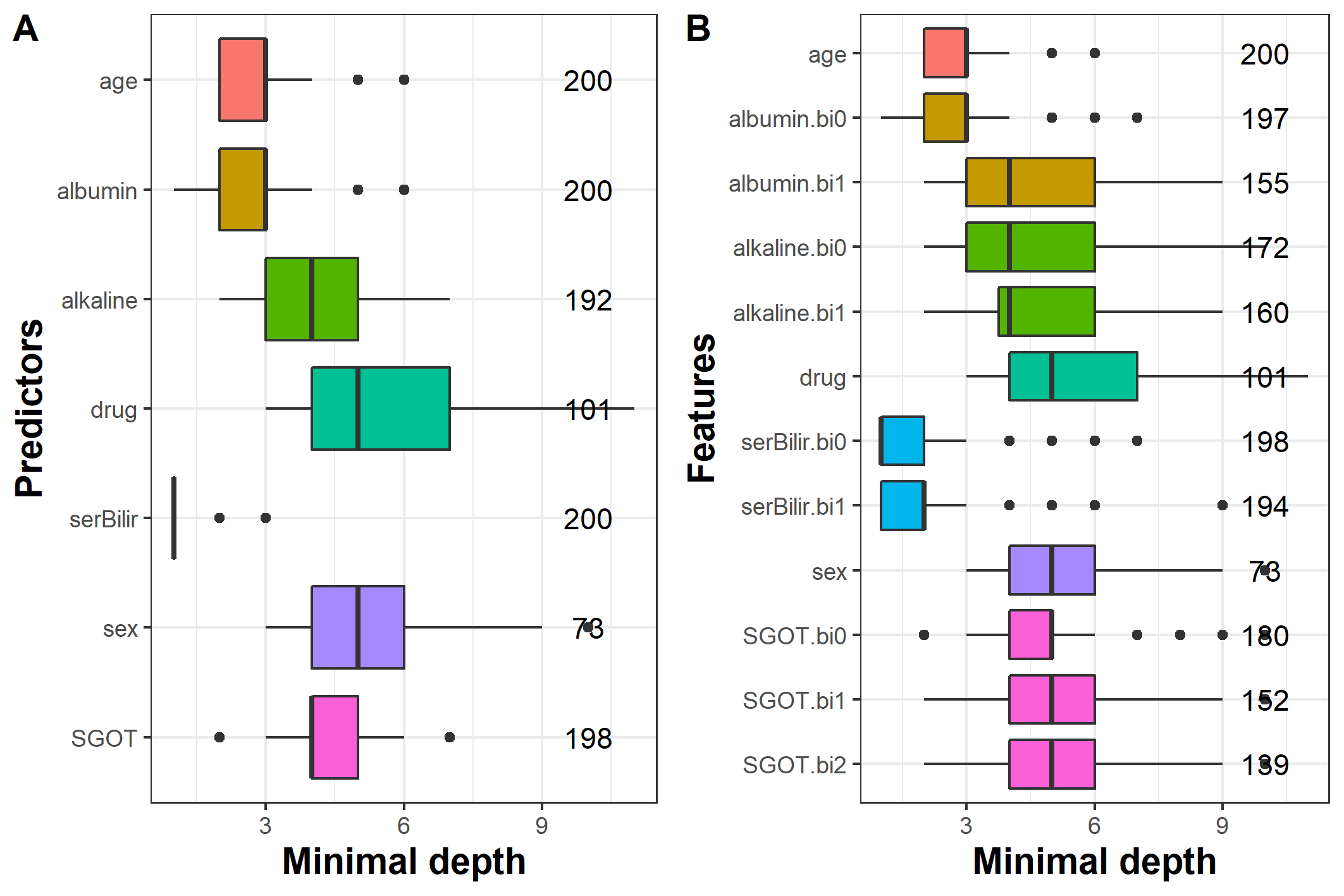Figure 4: Average minimal depth level by predictor (A) and feature (B).

## Guidelines to tune the hyperparameters

The predictive performance of the random forest strongly depends on the hyperparameters mtry, nodesize and minsplit, and should therefore be chosen thoroughly. nodesize and minsplit hyperparameters control the tree depth. The trees need to be deep enough to ensure that the predictions are accurate. By default in DynForest() function, we fixed nodesize = 1 and minsplit = 2, being the minimum. However, with a large number of individuals, the tree depth could be slightly decreased by increasing these hyperparameters to reduce the computation time.

mtry hyperparameter defines the number of predictors randomly drawn at each node. By default, we chose mtry equal to the square root of the number of predictors as usually recommended (Bernard, Heutte, and Adam 2009). However, this hyperparameter should be carefully tuned with possible values between 1 and the number of predictors. Indeed, the predictive performance of the random forest is highly related to this hyperparameter.

In the illustration, we tuned mtry for every possible values (1 to 7). The figure 5 displays the evolution of the OOB error according to mtry hyperparameter.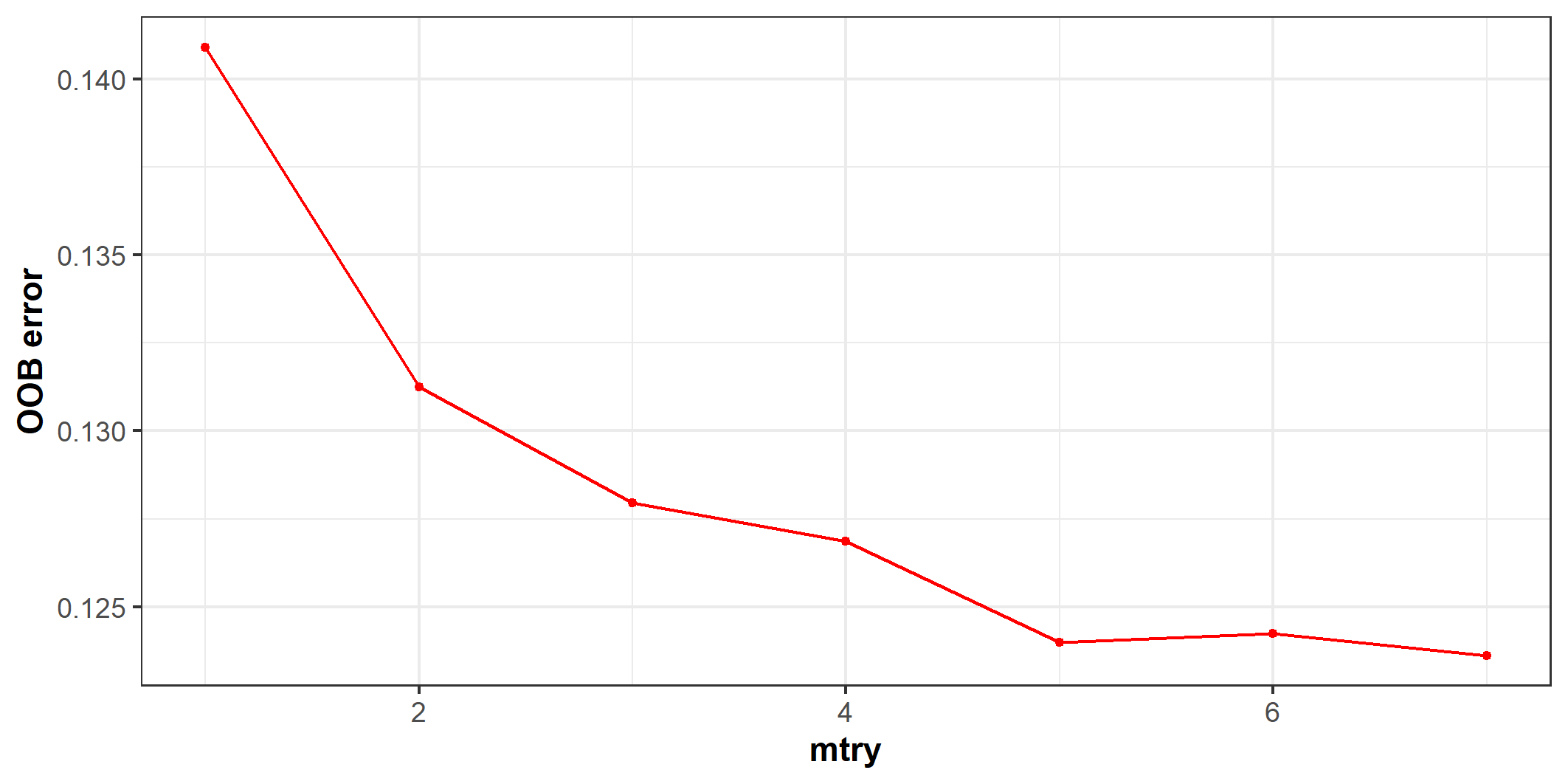Figure 5: OOB error according to mtry hyperparameter. The optimal value was found for mtry = 7.

We can see on this figure large OOB error difference according to mtry hyperparameter. In particular, we observe the worst predictive performance for lower values, then similar results with values from 5 to 7. The optimal value (i.e. with the lowest OOB error) was found with mtry = 7. This graph reflects how it is crucial to carefully tune this hyperparameter.